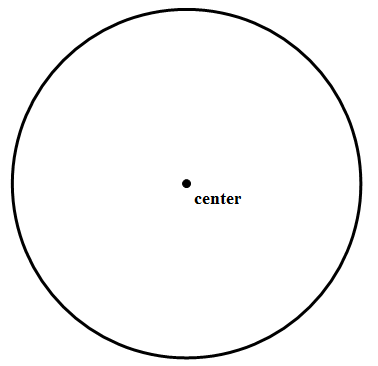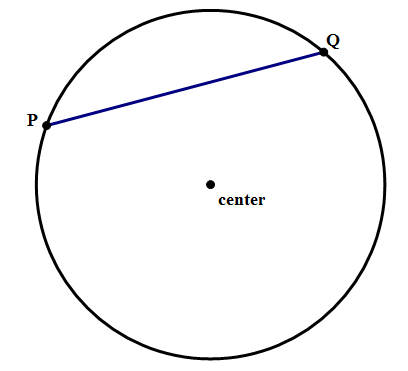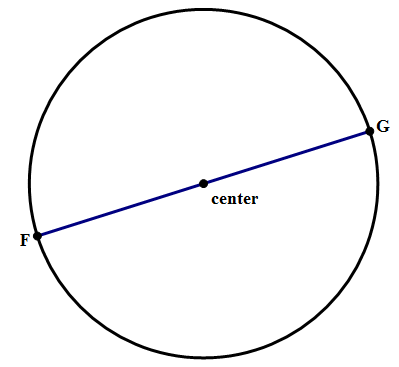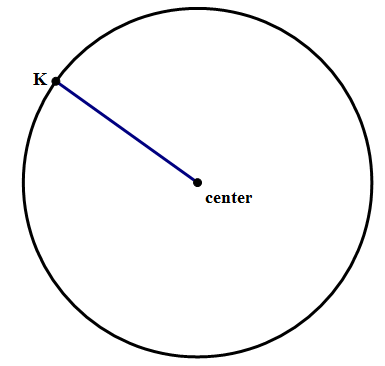# GMAT Quant: Geometry – Circles

Quantitative section of GMAT Exam consists of total 37 questions. The time allotted for this section is 75 minutes. There are two main question types:

Circle is the first geometric figure which we started playing with even before we knew the concepts of geometry.

What is a Circle?

A set of points, placed at equal distance from some fixed point, is known as a circle.The fixed point is known as a circle.

So, let’s understand some concepts related to circle:

1. Chord:Any segment of line that has its end points on the circle.

1. Diameter

A chord passing through center is known as a diameter.A radius is a line segment whose one end point lies at the center while other lies on the circle.Formulas related to circle:

Area of circle is equal to:

Perimeter of circle is:

Where, r is radius and d is diameter.

Let’s solve a question to get a better grasp at the concept:

Question:

What is the diameter of the circle which circumscribes a triangle with sides 9-40-41 cm?

Solution:As, 9-40-41 is a Pythagorean triplet. So, the hypotenuse of the triangle will be the diameter of the circle.

So, Diameter = 41 cm.

BYJU’S will be glad to help you in your GMAT preparation journey. You can ask for any assistance related to GMAT and MBA from us by calling us at +918884544444. You can write to us at gmat@byjus.com.